Home

# A Note on Algebras that are Sums of Two Subalgebras

## Abstract

We study an associative algebra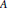$A$ over an arbitrary field that is a sum of two subalgebras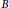$B$ and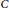$C$ (i.e.,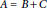$A\,=\,B+C$ ). We show that if$B$ is a right or left Artinian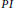$PI$ algebra and$C$ is a$PI$ algebra, then$A$ is a$PI$ algebra. Additionally, we generalize this result for semiprime algebras$A$ . Consider the class of all semisimple finite dimensional algebras$A\,=\,B+C$ for some subalgebras$B$ and$C$ that satisfy given polynomial identities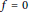$f\,=\,0$ and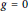$g\,=\,0$ , respectively. We prove that all algebras in this class satisfy a common polynomial identity.

## References

Hide All
MathJax
MathJax is a JavaScript display engine for mathematics. For more information see http://www.mathjax.org.

# A Note on Algebras that are Sums of Two Subalgebras

## Metrics

### Full text viewsFull text views reflects the number of PDF downloads, PDFs sent to Google Drive, Dropbox and Kindle and HTML full text views.

Total number of HTML views: 0
Total number of PDF views: 0 *Loading metrics...

### Abstract viewsAbstract views reflect the number of visits to the article landing page.

Total abstract views: 0 *Loading metrics...

* Views captured on Cambridge Core between <date>. This data will be updated every 24 hours.

Usage data cannot currently be displayed